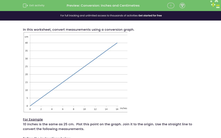# Conversion: Inches and Centimetres

In this worksheet, students convert given measurements (from inches to cm or cm to inches) using readings from a conversion graph.Key stage:  KS 2

Curriculum topic:   Measurement

Curriculum subtopic:   Convert Between Standard Units

Difficulty level:#### Worksheet Overview

In this worksheet, convert measurements using a conversion graph.For Example

10 inches is the same as 25 cm.  Plot this point on the graph. Join it to the origin. Use the straight line to convert the following measurements.

Plot this point on the graph.
Join it to the origin (0,0)
Use it to convert these measurements:

Question:

25 cm  = ___________ inches

15 cm  = ___________ inches